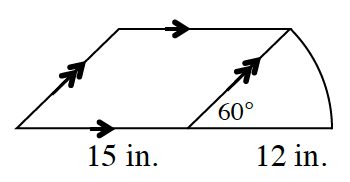### Home > CCG > Chapter 10 > Lesson 10.1.1 > Problem10-9

10-9.

A sector is attached to the side of a parallelogram, as shown in the diagram at right. Find the area and perimeter of the figure.

Find the arc length of the sector to help find the perimeter.

$\text{Arc length}=2\left(12\right)\pi\left(\frac{1}{6}\right)=\frac{24\pi}{6}$

$\text{Perimeter}=4\pi+27+12+15\approx66.6$